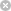RTXI  2.1Go to the documentation of this file.
1 //
3 //
4 #include <fstream>
5 #include <math.h>
6 #include <stdlib.h>
7 #include <iostream>
8
10 #include "dirform1.h"
11
12 #ifdef _DEBUG
13 extern std::ofstream DebugFile;
14 #endif
15
16 using namespace std;
17
19  long coeff_quan_factor, long input_quan_factor,
20  int tap_for_trans, int secondary_tap,
21  int transient_len)
22  : DirectFormFir(num_taps, coeff, quan_enab, coeff_quan_factor,
23  input_quan_factor)
24 {
25  int samp_idx;
26  Update_Count = 0;
27  Trial_Count = 0;
28  Tap_For_Trans = tap_for_trans;
29  Secondary_Tap = secondary_tap;
30  Transient_Len = transient_len;
31  Tally_For_Avg = new double[transient_len];
32  Tally_For_Avg_2 = new double[transient_len];
33  Sample_Transient = new double[transient_len];
34  Sample_Trans_2 = new double[transient_len];
35  for (samp_idx = 0; samp_idx < transient_len; samp_idx++) {
36  Tally_For_Avg[samp_idx] = 0.0;
37  Tally_For_Avg_2[samp_idx] = 0.0;
38  Sample_Transient[samp_idx] = 0.0;
39  Sample_Trans_2[samp_idx] = 0.0;
40  }
41 #ifdef _DEBUG
42  DebugFile << "In AdaptiveFir" << std::endl;
43 #endif
44  return;
45 }
46
48 {
49  delete[] Tally_For_Avg;
50  delete[] Tally_For_Avg_2;
51  delete[] Sample_Transient;
52  delete[] Sample_Trans_2;
53 }
54
55 void
57 {
58  int samp_idx;
59
60  ofstream trans_file("avg_tran.txt", ios::out);
61  for (samp_idx = 0; samp_idx < Transient_Len; samp_idx += decim_factor) {
62  trans_file << samp_idx << ", "
63  << (Tally_For_Avg[samp_idx] / double(Trial_Count)) << ", "
64  << Sample_Transient[samp_idx] << std::endl;
65  }
66  trans_file.close();
67 }
68 void
70 {
71  int samp_idx;
72  double x, y, old_x, old_y, dist;
73  ofstream trans_file("traject.txt", ios::out);
74
75  old_x = Sample_Transient;
76  old_y = Sample_Trans_2;
77  trans_file << (0) << ", " << old_x << ", " << old_y << std::endl;
78
79  for (samp_idx = 1; samp_idx < Transient_Len; samp_idx++) {
80  x = Sample_Transient[samp_idx];
81  y = Sample_Trans_2[samp_idx];
82  dist = sqrt((old_x - x) * (old_x - x) + (old_y - y) * (old_y - y));
83  if (dist < min_dist)
84  continue;
85  trans_file << samp_idx << ", " << x << ", " << y << std::endl;
86  old_x = x;
87  old_y = y;
88  }
89  trans_file.close();
90 }
91 void
93 {
94  int samp_idx;
95  double x, y, old_x, old_y, dist;
96  ofstream trans_file("avg_traj.txt", ios::out);
97
98  old_x = Tally_For_Avg / double(Trial_Count);
99  old_y = Tally_For_Avg_2 / double(Trial_Count);
100  trans_file << (0) << ", " << old_x << ", " << old_y << std::endl;
101
102  for (samp_idx = 1; samp_idx < Transient_Len; samp_idx++) {
103  x = Tally_For_Avg[samp_idx] / double(Trial_Count);
104  y = Tally_For_Avg_2[samp_idx] / double(Trial_Count);
105  dist = sqrt((old_x - x) * (old_x - x) + (old_y - y) * (old_y - y));
106  if (dist < min_dist)
107  continue;
108  trans_file << samp_idx << ", " << x << ", " << y << std::endl;
109  old_x = x;
110  old_y = y;
111  }
112  trans_file.close();
113 }
114 void
116 {
117  int samp_idx;
118
119  ofstream trans_file("samptran.txt", ios::out);
120
121  for (samp_idx = 0; samp_idx < Transient_Len; samp_idx += decim_factor) {
122  trans_file << samp_idx << ", " << Sample_Transient[samp_idx] << std::endl;
123  }
124  trans_file.close();
125 }
126 void
128 {
129  int i;
130  for (i = 0; i < Num_Taps; i++) {
131  taps[i] = Unquan_Coeff[i];
132  }
133 }
int Tap_For_Trans
void GetTaps(double *taps)
int logical
Definition: typedefs.h:4
AdaptiveFir(int num_taps, double *coeff, logical quan_enab, long coeff_quan_factor, long input_quan_factor, int tap_for_trans, int secondary_tap, int transient_len)
int Update_Count
double * Sample_Trans_2
int Transient_Len
complex sqrt(const complex _z)
Definition: complex.h:148
int Num_Taps
Definition: dirform1.h:21
int Trial_Count
double * Unquan_Coeff
Definition: dirform1.h:25
void DumpTransient(int decim_factor)
double * Sample_Transient
void DumpAvgTrajectory(double min_dist)